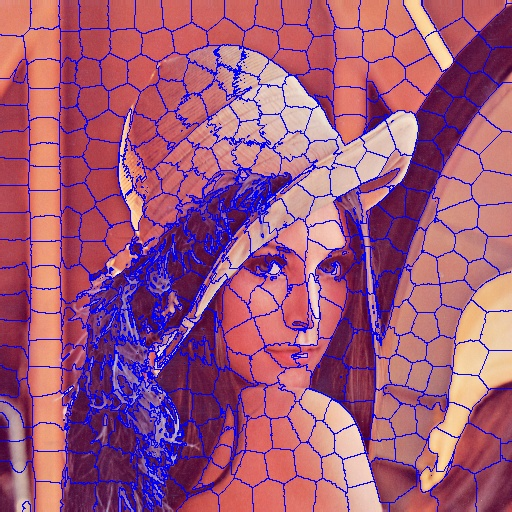Taskito - Calendar based to-do listIt’s been two months since I have written about my work. Well, to be honest, I didn’t do that much work which could be put up on a blog. I am working on a 3D application which is based on Android and when I get a chance, I’ll post about it. Meanwhile, I am also working with SimpleCV as part of my Google Summer of Code 2013 prgoram. For the mid term, I was working on porting it to cv2 and cleaning up the legacy code. I need to write a post about all the updates and new features that it will have. One of the features that I am planning to add is Superpixels based on SLIC algorithm.

What is a superpixel?

A superpixel can be defined as a group of pixels which have similar characteristics. It is generally color based segmentation. Superpixels can be very helpful for image segmentation. There are many algorithms available to segment superpixels but the one that I am using is state of the art with a low computational overhead.SLIC

Simple Linear Iterative Clustering is the state of the art algorithm to segment superpixels which doesn’t require much computational power. In brief, the algorithm clusters pixels in the combined five-dimensional color and image plane space to efficiently generate compact, nearly uniform superpixels. This algorithm was developed at Image and Visual Representation Group (IVRG) at EPFL and here’s the published paper and official source code.

How does SLIC work?

The approach is really simple actually. SLIC performs a local clustering of pixels in 5-D space defined by the L, a, b values of the CIELAB colorspace and x, y coordinates of the pixels. It has a different distance measurement which enables compactness and regularity in the superpixel shapes, and can be used on grayscale images as well as color images.

SLIC generates superpixels by clustering pixels based on their color similarity and proximity in the image plane. A 5 dimensional [labxy] space is used for clustering. CIELAB color space is considered as perpetually uniform for small color distances. It is not advisable to simply use Euclidean distance in the 5D space and hence the authors have introduced a new distance measure that considers superpixels size.

Distance Measure

SLIC takes a desired number of approximately equally-sized superpixels K as input. So each superpixels will have approximately N/K pixels. Hence, for equally sized superpixels, there would be a superpixel center at every grid interval S = √(N/K)

K superpixel cluster centers Ck = [lk, ak, bk, xk, yk] with k = [1, K] at regular grid intervals S are chosen. Since the spatial extent of any cluster is approximately S2, it can be assumed that pixels associated with this cluster lie within 2S x 2S area around the superpixel center in the xy plane.

Euclidean distances in CIELAB colorspace are meaningful for small distances. If spatial pixel distances exceed this perceptual color distance limit, then they begin to outweigh pixel color similarities.

Distance measure Ds is defined as follows.

dlab = √( (lk - li)2 + (ak - ai)2 + (bk - bi)2 )

dxy = √( (xk - xi)2 + (yk - yi)2 )

Ds = dlab + (m / S)* dxy

where Ds is the sum of the lab distance and the xy plane distance normalized by the grid interval S. A variable m is introduced in Ds allowing us to control the compactness of superpixel. The greater the value of m, the more spatial proximity is emphasized and the more compact the cluster. This value can be in the range [1, 20]. Authors of the algorithm have chosen m=10.

Algorithm

It begins by sampling K regularly spaced cluster centers and moving them to seed locations corresponding to the lowest gradient position in a 3 × 3 neighborhood. This is done to avoid placing them at an edge and to reduce the chances of choosing a noisy pixel. Image gradients are computed as

G(x, y) = || I(x + 1, y) − I(x − 1, y) ||2 + || I(x, y + 1) − I(x, y − 1) ||2

where I(x, y) is the lab vector corresponding to the pixel at position (x, y), and ||.|| is the L2 norm. This takes into account both color and intensity information.

Each pixel in the image is associated with the nearest cluster center whose search area overlaps this pixel. After all the pixels are associated with the nearest cluster center, a new center is computed as the average labxy vector of all the pixels belonging to the cluster.

At the end of this process, a few stray labels may remain, that is, a few pixels in the vicinity of a larger segment having the same label but not connected to it. It enforces connectivity in the last step of the algorithm by relabeling disjoint segments with the labels of the largest neighboring cluster.

Repository

Note: My implementation is based on PSMM implementation (above C++ code). Although, it works really well, Python implementation is slower and takes a lot of time for enforcing connectivity and drawing contours. I am still optimizing it.

P.S. Things are great.

Playing around with Android UI

Articles focusing on Android UI - playing around with ViewPagers, CoordinatorLayout, meaningful motions and animations, implementing difficult customized views, etc.